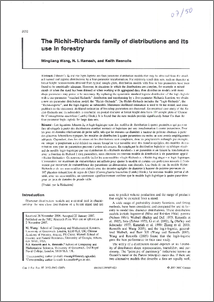# The Richit-Richards family of distributions and its use in forestry

Wang, Mingliang, Ramesh, Nadarajah I. and Rennolls, Keith (2007) The Richit-Richards family of distributions and its use in forestry. Canadian Journal of Forest Research, 37 (10). pp. 2052-2062. ISSN 0045-5067 (doi:https://doi.org/10.1139/X07-023)Preview
PDF
07_50.pdf

## Abstract

Johnson's SB and the logit-logistic are four-parameter distribution models that may be obtained from the standard normal and logistic distributions by a four-parameter transformation. For relatively small data sets, such as diameter at breast height measurements obtained from typical sample plots, distribution models with four or less parameters have been found to be empirically adequate. However, in situations in which the distributions are complex, for example in mixed stands or when the stand has been thinned or when working with aggregated data, then distribution models with more shape parameters may prove to be necessary. By replacing the symmetric standard logistic distribution of the logit-logistic with a one-parameter “standard Richards” distribution and transforming by a five-parameter Richards function, we obtain a new six-parameter distribution model, the “Richit-Richards”. The Richit-Richards includes the “logit-Richards”, the “Richit-logistic”, and the logit-logistic as submodels. Maximum likelihood estimation is used to fit the model, and some problems in the maximum likelihood estimation of bounding parameters are discussed. An empirical case study of the Richit-Richards and its submodels is conducted on pooled diameter at breast height data from 107 sample plots of Chinese fir (Cunninghamia lanceolata (Lamb.) Hook.). It is found that the new models provide significantly better fits than the four-parameter logit-logistic for large data sets.

Item Type: Article logit-logistics, four-parameter, six-parameter, Richit Richards Q Science > QA Mathematics > QA75 Electronic computers. Computer scienceS Agriculture > SD Forestry School of Computing & Mathematical SciencesSchool of Computing & Mathematical Sciences > Department of Mathematical SciencesSchool of Computing & Mathematical Sciences > Department of Computer ScienceSchool of Computing & Mathematical Sciences > Statistics & Operational Research Group 14 Oct 2016 09:03 http://gala.gre.ac.uk/id/eprint/1114View Item